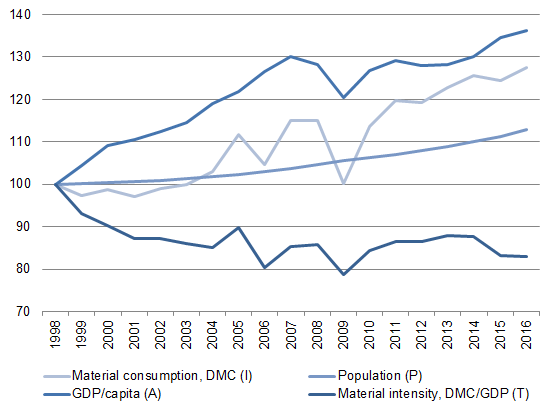To main content

# Driving factors for material consumption in Sweden according to the IPAT equation, 1998-2016

Index (1998 = 100)## Comments

The development of the domestic material consumption (DMC) can be considered to be the result of the development in three different factors:

- The amount of material needed per product, i.e., the material consumption per unit of GDP, the material intensity (DMC/GDP) (T)
- The amount of products produced per person (GDP/capita) (A)
- The amount of people living in a country (population) (P).

These factors multiplied, result in the total material consumption (DMC) for the country in question. This decomposition is known as the IPAT equation, where I (environmental Impact) = P (Population) * A (Affluence) * T (Technology). Here we have the material consumption DMC as the environmental impact (I), and the equation then reads I (DMC) = P (population) * A (GDP/capita) * T (DMC/GDP). In the diagram above, these factors have been normalized to index numbers.

## More about the results

Source file
Download as Excel

Source
SCB

Last updated
2018-12-12